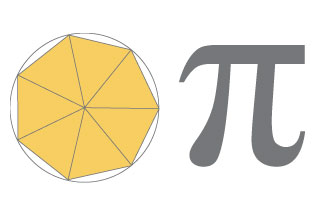# Approximating Pi

• By Rick Groleau
• Posted 09.01.03
• NOVA

Around 250 B.C., the Greek mathematician Archimedes calculated the ratio of a circle's circumference to its diameter. A precise determination of pi, as we know this ratio today, had long been of interest to the ancient Greeks, who strove for precise mathematical proportions in their architecture, music, and other art forms. Close approximations of pi had been known for over 1,000 years. Archimedes' value, however, was not only more accurate, it was the first theoretical, rather than measured, calculation of pi. Here, try Archimedes' approach yourself.The Greek mathematician Archimedes used a fairly simple geometrical approach to estimate pi. See how he did it.

This feature originally appeared on the site for the NOVA program Infinite Secrets.

### More on the method

Archimedes' method finds an approximation of pi by determining the length of the perimeter of a polygon inscribed within a circle (which is less than the circumference of the circle) and the perimeter of a polygon circumscribed outside a circle (which is greater than the circumference). The value of pi lies between those two lengths.

By doubling the number of sides of the hexagon to a 12-sided polygon, then a 24-sided polygon, and finally 48- and 96-sided polygons, Archimedes was able to bring the two perimeters ever closer in length to the circumference of the circle and thereby come up with his approximation.

Specifically, he determined that pi was less than 3 1/7 but greater than 3 10/71. In the decimal notation we use today, this translates to 3.1429 to 3.1408. That's pretty close to the known value of 3.1416. (For simplicity's sake, we round off all figures to four decimal places.)

Like Archimedes' approach, our interactive doesn't rely on specific measurements. The diameter of the circle is given an arbitrary value of 1; it doesn't matter if that number represents an inch, a foot, or a light-year. Also like Archimedes' approach, the interactive determines the length of a side of each triangle, relative to the diameter, based on the angle opposing the side being measured.

Our interactive differs from Archimedes' approach in three key ways, however. First, it makes use of algebra and modern trigonometry, which were unknown in Archimedes' day—Archimedes used geometry instead. For example, he knew the ratio between one line and another in certain triangles and with this knowledge was able to figure out the length of the perimeter of a hexagon.

Second, we use decimal notation, which wasn't invented until hundreds of years after Archimedes' death. To work with non-whole numbers, the ancients relied on ratios. Any calculator will tell you that the square root of 3 is 1.7321. For Archimedes, that value was 265/153 (which equals 1.7320 in decimal notation).

Finally, our interactive increases the number of sides of the hexagon to 96 by increments of 1 rather than by the doublings Archimedes used. The idea is to give you a clearer sense of how ever closer in length to the perimeter of the circle the length of the hexagonal perimeters becomes with each added side.

It is interesting to note that even today pi cannot be calculated precisely—there are no two whole numbers that can make a ratio equal to pi. Mathematicians find a closer approximation every year—in 2002, for example, experts at the University of Tokyo Information Technology Center determined the value of pi to over one trillion decimal places. But this is academic: the value determined by Archimedes over 2,000 years ago is sufficient for most uses today.

(all)